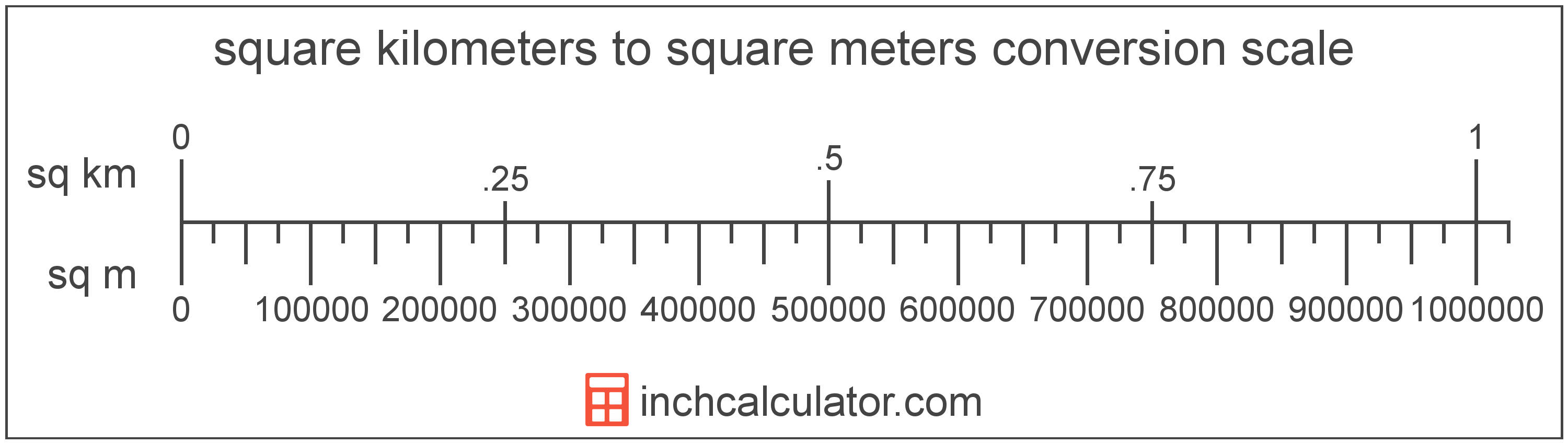# Square Kilometers to Square Meters Converter

Enter the area in square kilometers below to get the value converted to square meters.

Results in Square Meters:1 sq km = 1,000,000 sq m

Do you want to convert square meters to square kilometers?

## How to Convert Square Kilometers to Square Meters

To convert a measurement in square kilometers to a measurement in square meters, multiply the area by the following conversion ratio: 1,000,000 square meters/square kilometer.

Since one square kilometer is equal to 1,000,000 square meters, you can use this simple formula to convert:

square meters = square kilometers × 1,000,000

The area in square meters is equal to the area in square kilometers multiplied by 1,000,000.

For example, here's how to convert 5 square kilometers to square meters using the formula above.
square meters = (5 sq km × 1,000,000) = 5,000,000 sq m### How Many Square Meters Are in a Square Kilometer?

There are 1,000,000 square meters in a square kilometer, which is why we use this value in the formula above.

1 sq km = 1,000,000 sq m

## What is a Square Kilometer?

One square kilometer is equivalent to the area of a square with sides that are each 1 kilometer in length. One square kilometer is equal to 0.386102 square miles or 1,000,000 square meters.

The square kilometer, or square kilometre, is a multiple of the square meter, which is the SI derived unit for area. In the metric system, "kilo" is the prefix for thousands, or 103. A square kilometer is sometimes also referred to as a square km. Square kilometers can be abbreviated as sq km, and are also sometimes abbreviated as km². For example, 1 square kilometer can be written as 1 sq km or 1 km².

## What is a Square Meter?

One square meter is equivalent to the area of a square with sides that are each 1 meter in length. One square meter is equal to 10.76391 square feet or 10,000 square centimeters.

The square meter, or square metre, is the SI derived unit for area in the metric system. A square meter is sometimes also referred to as a square m. Square meters can be abbreviated as sq m, and are also sometimes abbreviated as . For example, 1 square meter can be written as 1 sq m or 1 m².

You can use a square meters calculator to calculate the area of a space if you know its dimensions.

## Square Kilometer to Square Meter Conversion Table

Table showing various square kilometer measurements converted to square meters.
Square Kilometers Square Meters
0.000001 sq km 1 sq m
0.000002 sq km 2 sq m
0.000003 sq km 3 sq m
0.000004 sq km 4 sq m
0.000005 sq km 5 sq m
0.000006 sq km 6 sq m
0.000007 sq km 7 sq m
0.000008 sq km 8 sq m
0.000009 sq km 9 sq m
0.0000001 sq km 0.1 sq m
0.000001 sq km 1 sq m
0.00001 sq km 10 sq m
0.0001 sq km 100 sq m
0.001 sq km 1,000 sq m
0.01 sq km 10,000 sq m
0.1 sq km 100,000 sq m
1 sq km 1,000,000 sq m

## References

1. Collins Dictionary, Definition of 'square meter', https://www.collinsdictionary.com/us/dictionary/english/square-meter